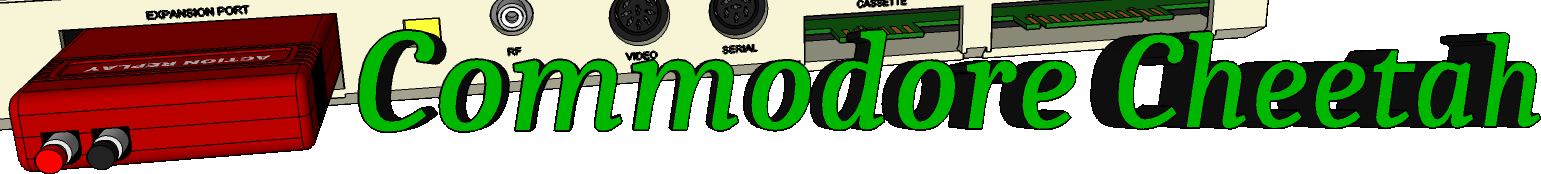Home PageeMail
email5-commodorecheetah.co.uk

Note: You will need to replace the '-' with the @ symbol.
This is to minimize spam.

 My BASIC Quick Reference Guide DEF Abbreviation: D TYPE: Statement FORMAT: DEF FN ( ) = Action: This sets up a user-defined function that can be used later in the program. The function can consist of any mathematical formula. User defined functions save space in programs where a long formula is used in several places. The formula need only be specified once, in the definition statement, and then it is abbreviated as a function name. It must be executed once, but any subsequent executions are ignored. The function name is the letters FN followed by any variable name. This can be 1 or 2 characters, the first being a letter and the second a letter or digit. EXAMPLES of DEF FN Statement: 10 DEF FN A(X)=X+7 20 DEF FN AA(X)=Y*Z 30 DEF FN A9(Q) = INT(RND(1)*Q+1) The function is called later in the program by using the function name with a variable in parentheses. This function name is used like any other variable, and its value is automatically calculated, EXAMPLES of FN Use: 40 PRINT FN A(9) 50 R=FN AA(9) 60 G=G+FN A9(10) In line 50 above, the number 9 inside the parentheses does not affect the outcome of the function, because the function definition in line 20 doesn't use the variable in the parentheses. The result is Y times Z, regardless of the value of X. In the other two functions, the value in parentheses does affect the result.

Commodore Cheetah made by Allen Monks, started in the year 2000.